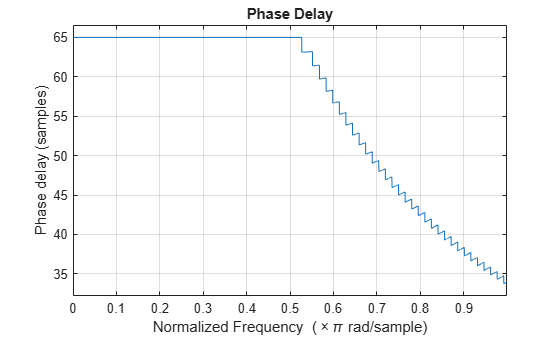phasedelay

Phase delay response of discrete-time filter System object

Description

[phi,w] = phasedelay(sysobj) returns the phase delay response vector, phi, and the corresponding frequency vector, w, in radians/sample of the filter System object™ based on the current filter coefficients.

[phi,w] = phasedelay(sysobj,n) returns the n-point phase delay response vector and the corresponding n-point frequency vector in radians/sample of the filter System object.

[phi,w] = phasedelay(sysobj,n,fs) uses fs to calculate the delay response. When you specify this syntax without any output arguments, the function launches fvtool and plots the response to fs/2.

[phi,w] = phasedelay(___,'Arithmetic',arithType) analyzes the filter System object, based on the arithmetic specified in arithType using anyone of the previous syntaxes.

example

phasedelay(sysobj) launches fvtool and shows the phase delay response of the filter System object.

For more input options, see phasedelay in Signal Processing Toolbox™.

Examples

collapse all

Design an FIR filter.

Fs = 8000; Fcutoff = 2000;
FIRFilt = dsp.FIRFilter('Numerator', fir1(130,Fcutoff/(Fs/2)));

The phasedelay function computes the phase delay of the filter and displays it using FVTool.

phasedelay(FIRFilt)Input Arguments

collapse all

Number of points over which the phase response is evaluated around the upper half of the unit circle. For an FIR filter where n is a power of two, the computation is done faster using FFTs.

Data Types: double

Sampling frequency used in computing the phase delay response, specified as a positive scalar.

Data Types: single | double

Arithmetic used in the filter analysis, specified as 'double', 'single', or 'Fixed'. When the arithmetic input is not specified and the filter System object is unlocked, the analysis tool assumes a double-precision filter. When the arithmetic input is not specified and the System object is locked, the function performs the analysis based on the data type of the locked input.

The 'Fixed' value applies to filter System objects with fixed-point properties only.

When the 'Arithmetic' input argument is specified as 'Fixed' and the filter object has the data type of the coefficients set to 'Same word length as input', the arithmetic analysis depends on whether the System object is unlocked or locked.

• unlocked –– The analysis object function cannot determine the coefficients data type. The function assumes that the coefficients data type is signed, has a 16-bit word length, and is auto scaled. The function performs fixed-point analysis based on this assumption.

• locked –– When the input data type is 'double' or 'single', the analysis object function cannot determine the coefficients data type. The function assumes that the data type of the coefficients is signed, has a 16-bit word length, and is auto scaled. The function performs fixed-point analysis based on this assumption.

To check if the System object is locked or unlocked, use the isLocked function.

When the arithmetic input is specified as 'Fixed' and the filter object has the data type of the coefficients set to a custom numeric type, the object function performs fixed-point analysis based on the custom numeric data type.

Output Arguments

collapse all

Phase delay response vector, evaluated at n equally-spaced points around the upper half of the unit circle, returned as a vector. If n is not specified, the function uses a default value of 8192.

Data Types: double

Frequency vector of length n in radians/sample. w consists of n equally-spaced points around the upper half of the unit circle (from 0 to π radians/sample). If n is not specified, the function uses a default value of 8192.

Data Types: double